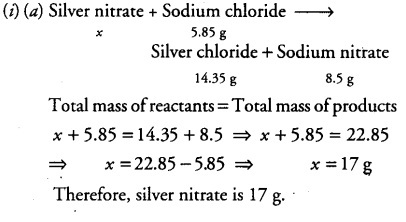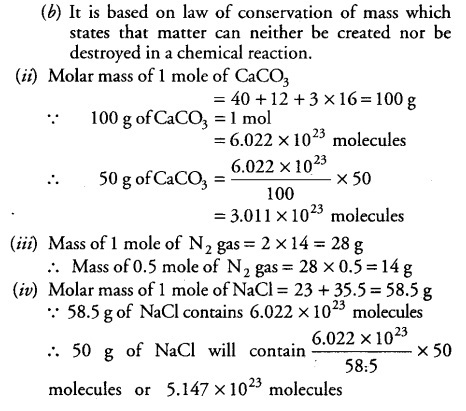# What mass of silver nitrate will react

(i) (a)What mass of silver nitrate will react

with 5.85 g of sodium chloride to produce 14.35 g of silver chloride and
8.5 g of sodium nitrate?
(b) On what law is the above reaction based and state the law?
(i) Calculate the number of molecules in 50 g of CaC03.
(iii) Calculate the mass of 0.5 mole of nitrogen gas.
(iv) Calculate the number of molecules in 50 g of NaCl.
[Atomic mass of Ca = 40 u, C = 12 u,
O = 16 u, N = 14u, Na = 23u, Cl = 35.5u and NA =6.022X \${10}B^{23}\$ mol-1]